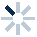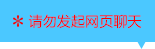| | |

||
APP端下载AndroidiPhone
|
￥140

|
1033人点赞
125408人已学习
|

3天无理由退款

3天无理由退款：退款将以超级币形式退至您的超级课堂学习账户，便于您重新选购其他课程。恶意退款将被冻结账号。

• 1、对数函数的概念，判断对数函数要注意三点：(1)底数$a$为大于$0$且不为$1$的常数。(2)真数位置上只能有$x$这一项。(3)整个对数式的系数必须是$1$，且后面不能有不为零的常数。对数函数的定义域为$(0,＋\infty )$。确定底数$a$可以采用待定系数法，但不能带入$(1,0)$，因为对数函数的图象一定经过点$(1,0)$
2、 $0＜a＜1$时，图象下降，函数递减。当$a＞1$时，图象上升，函数递增。
3、 图象都在$y$轴右侧，$y$轴是它们的渐近线，值域为$R$。且都经过$(1,0)$这个定点。若两个对数函数的底数互为倒数。它们的图象总关于$x$轴对称。
4、 对比指数函数的图象，它们的定义域和值域恰好相反。都过定点。且单调性受$a$影响的规律是一致的。即底数$0＜a＜1$,两种函数都是单减的；$a＞1$都是单增的
• 1、学习对数函数图象在题目中的应用
• 1、认识第一类绝对值函数的图象及常用规律
2、 第一类是套$x$的类型，图象左右对称。根据$a$的范围，分为喇叭口朝下和朝上两种图象。常见的还有它的平移变换后的函数，变换依旧遵循“左加右减”原则。对称轴为$x=-k$
• 1、认识第二类绝对值函数的图象及常用规律
2、 第二类是整体套类型，图象都为$v$型，顶点都为$(1,0)$。但要注意$a$会影响每段图象对应的解析式，在画图时，不妨也写出每段的解析式
• 1、学习两种套法相结合的绝对值函数。作图象时，按前面两种画法分步操作即可。图象为关于$x=-k$对称的断开的“$vv$”型
2、 两个顶点在$x$轴上，分别为$(-1-k,0)$和$(1-k,0)$
• 1、认识有关对数函数的第一类题型——由单调性求字母范围
2、 由函数递减推出$0＜a＜1$，由函数递增推出$a＞1$。涉及分段函数单调性满足的条件，以及通过图象判断字母参数的范围，要注意结对数函数的应用—对数函数的单调性与值域合二次函数的知识
• 1、认识有关对数函数的第二类题型——与值域相关的问题
2、 当底数固定时，根据单调性，结合图象就能求出相应区间内的值域
3、 当底数有未知字母时，若条件给出的是最值之和，则不需要准确知道哪个是最大值，哪个是最小值，故不需要分类讨论。若给出的是最值之差，或倍数关系，则需要搞清哪个是最大值，哪个是最小值，此时需要按底数分类讨论。
• 1、​对于第一类对数不等式，解法是将常数$b$化为以$a$为底数的对数$log_{a}a^{b}$，再根据对数函数$y=log_{a}x$的单调性来得到真数$f(x)$与$a^{b}$的大小关系，同时要注意真数$f(x)＞0$
• 1、对于第二类对数不等式$log_{a}f(x)＜log_{a}g(x)$，可以根据底数决定的外层对数函数的单调性，得到内层函数$f(x)$与$g(x)$的大小关系。不过要注意，真数都是要大于$0$的。所以一般会得到三个不等式组成的不等式组
2、 如果不等式两侧底数不同，就要进行化同底。如果两个对数的底数不能直接相互转化，就要把它们的底数转化成另外同一个数
• 1、对于第二类对数不等式，如果对数式的底数含有参数，就要分类讨论
2、 最后一道题要注意的是，在通过不等式组求交集时，要利用底数分类的前提条件
• 1、​对于第三类对数不等式，及可以化为这种形式的不等式，可以采用是换元法
2、 通过两边同时平方，化同底，取对数等处理，某些不等式都能变成第三类对数不等式的基本形式
• 1、讲解了一道第三类对数不等式的综合题，核心解法就在于对于$x^{log_{a}x}$这一项的处理，大家要记住对它取对数，从而把指数变成系数
• 1、对于含$x$的项较多，且无法用换元法化简的对数不等式，要尝试用图象法去解决
2、 把含对数式的项和其他类型的项分别放在不等号两边。观察出它们属于那种函数结构，通过图像的上下关系，来求不等式的解集
• 1、学习第一种解决不规则的指数不等式、对数不等式的方法——利用相似结构构造函数法
2、 通过移项，使不等号两侧结构相同，根据相似结构构造函数
• 1、学习第二种解决不规则指数不等式、对数不等式的方法——整体构造函数法
2、 通过每一项都除以同一个指数式，整体构造出一个单调性确定的函数
• 1、讲解一道综合利用构造函数法，解决不规则指数不等式、对数不等式的题目
2、 对于更加不规则的对数不等式，需要通过换元，恒等式等数学工具进行转化
• 1、介绍如何利用构造函数法，解决不规则的指数不等式、对数不等式
2、 可以通过移项，使不等号两侧结构相同，根据相似结构构造函数
3、 也可以通过每一项都除以同一个指数式，整体构造出一个单调性确定的函数
4、 对于更加不规则的对数不等式，需要通过换元，恒等式等数学工具进行转化
5、 最后一道题就是一个很典型的例子，同学们要好好揣摩这两大技巧和思维步骤
• 1、利用图象法和换底法解决第二类—底数不同，真数相同的对数式大小比较问题
2、 图象法的关键，是要记住底数和图象位置关系的规律。通过这个规律就能画出底数不同的对数函数图像的大致位置关系，再用一根代表相同真数的竖线，根据交点的上下关系，就能判断出对数值的大小关系。当然，也能反用，通过图像，判断底数的大小关系
3、 换底法，即把题目化为以同底数对数为分母的倒数的大小比较问题
• 1、学习处理底数、真数都不同的对数式的大小比较的第一种方法——标准值法
2、 对于标准值法，可以选取$0$或$\pm 1$作为标准值。同时要记住一个常用规律：如果底数与真数同大于$1$或同小于$1$，那么对数值大于$0$；如果底数与真数一个大于$1$，一个小于$1$，那么对数值小于$0$
• 1、学习处理底数、真数都不同的对数式的大小比较的第二种方法——图象法
2、 对于图象法，在同一坐标系中画出每个函数的图象，然后找真数对应的点，通过点的高低判断大小关系
3、 还额外介绍了独特的函数构造法。它的关键在于，要发现一系列对数式存在的代数结构上的规律
• 1、对数型复合函数求定义域依然遵行“由外向内”的原则。对于外层为对数函数的类型，首先要保证真数$f(x)$大于$0$，然后再考虑内层函数$f(x)$的定义域。切忌随意合并原函数，否则会改变定义域。对于内层为对数函数的类型，首先考虑外层函数$y=f(u)$的定义域，求出$u$的范围，即$log_{a}x$的范围，再求出$x$的范围，得到定义域
2、 过定点的问题也非常简单，对于外层为对数函数的复合函数。若$f(m)=1$，则图象过定点$(m,0)$。对于内层为指数函数的复合函数，图象过定点$(1,f(0))$
• 1、对数型复合函数的单调性依然遵循“同增异减”的原则，千万不要忘记真数大于$0$的定义域的潜在限定
2、 对于组合型的对数型复合函数，先尝试根据组合函数单调性的规律来判断，如果行不通，就要进行合并，但要先求定义域，因为合并会改变原函数定义域
• 1、学习外层为对数函数的复合函数，$y=log_{a}f(x)$的值域求解技巧
2、 原理是先在定义域的基础上求出内层函数$y=log_{a}u$的值域，再将它作为外层对数函数的定义域，从而求出$y$的范围，即复合函数值域
• 1、学习一类常见题型：什么情况下$y=log_{a}f(x)$的定义域为$R$，什么情况下它的值域为$R$
2、 若定义域为$R$，真数$f(x)$必须恒大于$0$，反映在图象上就是$f(x)$图象完全在$x$轴上方
3、 若值域为$R$，则需要$(0，+∞)$是$u$的子集，即$f(x)$值域的子集。反映在图象上，就是$f(x)$的值域要能覆盖$y$轴的正半轴
4、 如果把值域由$R$改成其他区间，处理方法也一样。注意，借助图象研究会更直观
• 1、讲解一道对数型复合函数的单调性与值域相结合的问题，同学们要认真体会涉及到的解题技巧
• 1、学习内层为对数函数的复合函数的值域求解技巧，基本方法还是和普通复合函数求值域的方法一样，结合图象去分析
2、 需要注意使用的技巧主要有：对数式化同底，和含参
• 1、讲解一道含参数和化同底这两个技巧相结合的题目，同学们要认真体会各种技巧的巧妙使用
• 1、讲解一道极难的综合题，这一道题含金量很高，体现了非常严谨的数学思维，同学们要注意体会

• 1小新卖蜡笔
• 2超课牛皮
• 3超级学员
• 4超级学员1611235
• 5超级学员2065910
• 6超级学员2627305
• 7超级学员3113891
• 8超级学员3742444
• 9超级学员4117693
• 10超级学员4156028196492人在学
￥ 78 ￥ 78127166人在学
￥ 78 ￥ 78219569人在学
￥ 0 ￥ 0126349人在学
￥ 72 ￥ 72

• 0
• 点击分享有好礼
••app端下载关注微信号